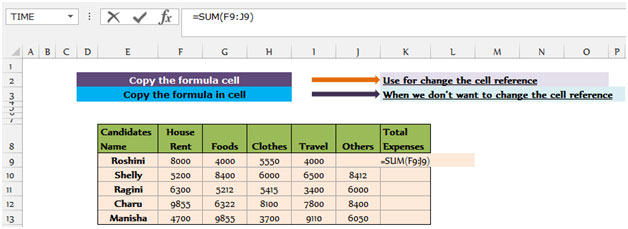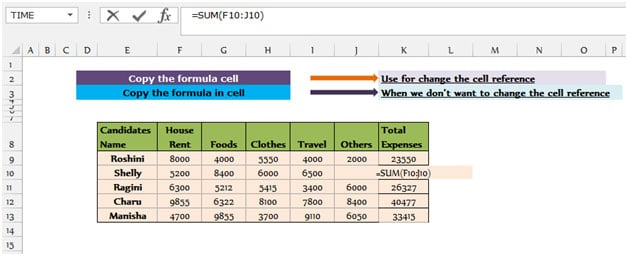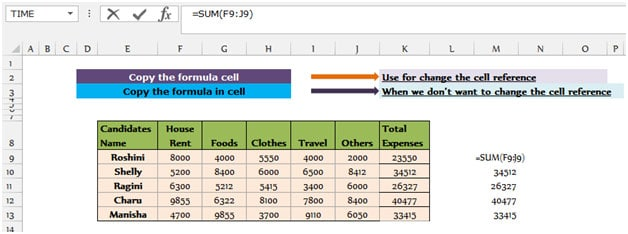# Simply put, when we copy the formula from cell to cell, the Draxel cell reference changes. However, when we transfer each formula from cell to cell, the cell reference will be the same.

In this article we will learn how to copy the formula from one range or cell to another range or cell in Excel.

In other words, we can copy the formulas in two ways and get different results.

Let’s take an example to better understand how we can copy functions in two different ways.

We have a table from ” E8 to K13 ” that details the monthly expenses of the candidates. The first row of the table ( E8 to K8 ) contains the title, the first column ” E ” contains the names of the candidates and the ” K ” column contains the total costs. We put the sum formula to return the total cost to the first candidate in cell K9 .## Change the cell reference

Follow the steps below to get the total cost for the other candidates:

Copy cell K9 by pressing “CTRL + C” and place it on your keyboard in the range K10 to K13 using “CTRL + V” .

Cell K9 basically contains the sum of the costs for row 9, when we copy the formula and put it in the bottom cells, the range reference changes automatically and we do not need to change it manually.## Copy the exact formula:

After obtaining the sum of costs for all candidates in the range of “K9 to K13” by copying the formula, we now do not want the amount to remain in the range of “K9 to K13” and we want to move its position to “M9 to” M13 ” change. If you copy the range “K9 to K13” by pressing CTRL + C and paste it in the range “M9 to M13” by pressing CTRL + V , you will see that the reference range “F9 to J9” to “H9 to L9” “It changes, and we do not want that to happen.

To reach the actual range, which is “F9 to J9” , the actual range remains the same when changing the position of the Total Expenses column .

• We must first select the formula cell.
• Press the F2 key , copy the formula using the Ctrl + C key and paste it on your keyboard in cell M9 using the Ctrl + V key .
• We will see that the references to the sum of the values ​​are still the same as in cell K9 .
• Copy cell M9 by pressing “CTRL + C” and place it in the range K10 to K13 using “CTRL + V” .So simply put, if we want the cell reference to change automatically, we have to use the copy function, and when we do not want the reference to change, we have to use the move function .